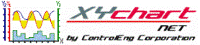XY Chart NET 3 Control Reference
SetZoomWindow Method

Use this method to perform a zoom to the specified min and max values.   This method is only available in the zoom mode (see SetZoomMode).

Syntax
 [Visual Basic] Sub SetZoomWindow(newXMin As Double, newXMax As Double, XScaleIndex As Integer, newYMin As Double, newYMax As Double, YScaleIndex As Integer) [C#] void XYChartNETCtl.SetZoomWindow(double newXMin, double newXMax, int XScaleIndex, double newYMin, double newYMax, int YScaleIndex) [C++] void SetZoomWindow(double, double, int, double, double, int)

Parameters
newXMin
Indicates the plot's new minimum x-value for the passed in x-scale.
newXMax
Indicates the plot's new maximum x-value for the passed in x-scale.
XScaleIndex
Indicates which x-axis the new min and max values pertain to.  For charts with multiple x-scales, all other scales are zoomed to its corresponding values.  Valid range: 0 to NumXScales - 1.
newYMin
Indicates the plot's new minimum y-value for the passed in y-scale.
newYMax
Indicates the plot's new maximum y-value for the passed in y-scale.
YScaleIndex
Indicates which y-axis the new min and max values pertain to.  For charts with multiple y-scales, all other scales are zoomed to its corresponding values.  Valid range: 0 to NumYScales - 1.
Example
```' XYChartNETCtl1 is the name of the XY Chart NET control instance placed on the form.

Dim xMin As Double
Dim xMax As Double
Dim yMin As Double
Dim yMax As Double
Dim xScaleIdx As Integer
Dim yScaleIdx As Integer

xMin = CDbl(XMinValue.Text)     'New x-min value
xMax = CDbl(XMaxValue.Text)     'New x-max value
xScaleIdx = 0                   'X-axis scale index the new x-min/max pertains to
yMin = CDbl(YMinValue.Text)     'New y-min value
yMax = CDbl(YMaxValue.Text)     'New y-max value
yScaleIdx = 0                   'Y-axis scale index the new y-min/max pertains to

'Perform a zoom; a Refresh call is not required
XyChartNETCtl1.SetZoomMode(True)
XyChartNETCtl1.SetZoomWindow(xMin, xMax, xScaleIdx, yMin, yMax, yScaleIdx)
```
```
```
```
```